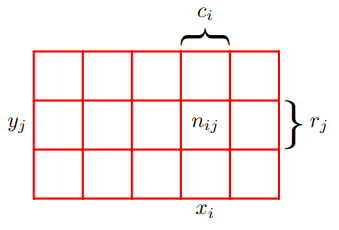# 第一章 介绍

## 1.1 例子：多项式曲线拟合

$E(w)=\frac{1}{2} \sum_{i=1}^N{(y(x_i, w)-t_i)^2}$

$E_{RMS}=\sqrt{\frac{2E(w)}{N}}$

$\stackrel{\sim}{E}=\frac{1}{2}\sum_{n=1}^N{\{y(x_n,w)-t_n\}^2}+\frac{\lambda}{2}||w||^2$ 其中$||w||^2=ww^T=w_0^2+w_1^2+\ldots+w_M^2$，系数$\lambda$用于调整正则项对结果影响的强度。一般$w_0$不会算在正则化子中，因为如果加入$w_0$，会导致误差依赖于选择的模型在$x=0$时所取的值，或则可以加入$w_0$，但是要分配一个独立的系数。在统计学中，这样的技术称为收缩方法，因为他们会减少系数的值。上面的二阶正则化子的特殊情况称为脊回归（ridge regression）。在神经网络上下文中，这种方法也称为权重衰退（weight decay）。

## 1.2 概率论$X$取$x_i$而$Y$取$y_j$的概率写作$p(X=x_i, Y=y_j)$，并称为$X=x_i$和$Y=y_j$的联合概率。因此 $p(X=x_i,Y=y_j)=\frac{n_{ij}}{N}$ 这里我们隐式地考虑了极限$N\rightarrow \infty$。同样，$X$取值$x_i$的概率写作$p(X=x_i)$，并以分数的形式给出，因此： $p(X=x_i)=\frac{c_i}{N}$ 由于落在第i列的事件数目，实际是落在第i列上各个单元格上事件数目的综合，因此我们有$c_i=\sum_j{n_{ij}}$，而通过上面两个公式可以得到 $p(X=x_i)=\sum_{j=1}^L{p(X=x_i,Y=y_j)}$ 注意$p(X=x_i)$有时也称作临界概率（marginal probability），因为它是通过加总其他随机变量得到的（这个例子中为$Y$）。

### 1.2.1 概率密度

$\int_{-\infty}^\infty{p(x)dx} = 1$

$x$落在区间$(-\infty,z)$的概率可以通过累计分布函数得到，其定义为： $P(z)=\int_{-\infty}^z{p(x)dx}$ 满足$P'(x)=p(x)$。如果我们有数个连续变量$x_1, \ldots , x_D$，记作向量$x$，那么我们可以定义联结概率密度$p(x)=p(x_1,\ldots ,x_D)$。而$x$落在无限小的空间$\delta x$中的概率由$p(x)\delta x$。多元概率密度必须满足 $p(x)\ge 0$

$\int{p(x)dx}=1$

$p(x,y)=p(y|x)p(x)$

### 1.2.2 期望和协方差

$\mathrm{E}[f]=\sum_x{p(x)f(x)}$ 对于连续变量的情况，期望有下面形式： $\mathrm{E}[f]=\int{p(x)f(x)dx}$ 无论哪种情况，如果我们按照概率分布给出有限的$N$个点，那么期望可以被接近于这些有限点的加总和： $\mathrm{E}[f]\backsimeq \frac{1}{N} \sum_{n=1}^N{f(x_n)}$ 上面的接近将在$N\rightarrow\infty$时将变成相等。